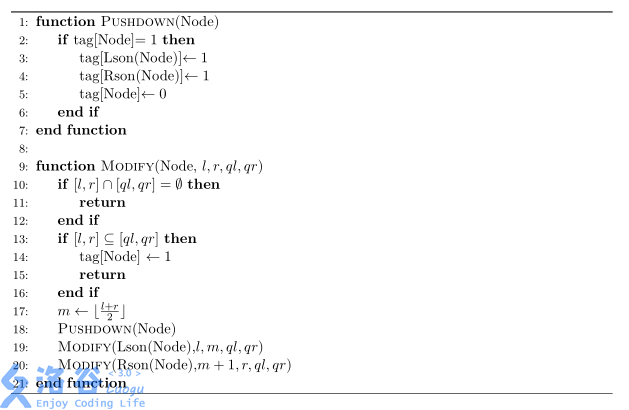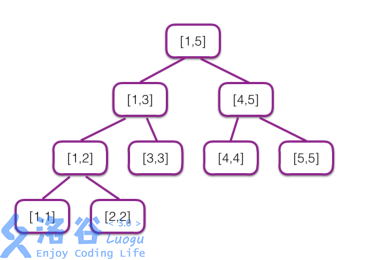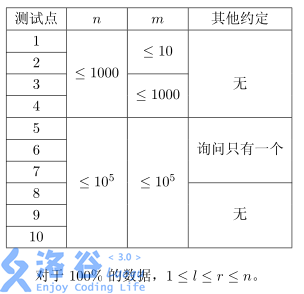P5280 [ZJOI2019]线段树

• 316通过
• 658提交
• 题目提供者 jiry_2
• 评测方式 云端评测
• 标签 各省省选 2019 浙江 O2优化
• 难度 NOI/NOI+/CTSC
• 时空限制 3000ms / 512MB
• 提示：收藏到任务计划后，可在首页查看。

题目描述

九条可怜是一个喜欢数据结构的女孩子，在常见的数据结构中，可怜最喜欢的就是线段树。

线段树的核心是懒标记，下面是一个带懒标记的线段树的伪代码，其中 $tag$ 数组为懒标记：其中函数 $\operatorname{Lson}(Node)$ 表示 $Node$ 的左儿子，$\operatorname{Rson}(Node)$ 表示 $Node$ 的右儿子。

现在可怜手上有一棵 $[1,n]$ 上的线段树，编号为 $1$。这棵线段树上的所有节点的 $tag$ 均为$0$。接下来可怜进行了 $m$ 次操作，操作有两种：

• $1\ l\ r$，假设可怜当前手上有 $t$ 棵线段树，可怜会把每棵线段树复制两份（$tag$ 数组也一起复制），原先编号为 $i$ 的线段树复制得到的两棵编号为 $2i-1$ 与 $2i$，在复制结束后，可怜手上一共有 $2t$ 棵线段树。接着，可怜会对所有编号为奇数的线段树进行一次 $\operatorname{Modify}(root,1,n,l,r)$。

• $2$，可怜定义一棵线段树的权值为它上面有多少个节点 $tag$ 为 $1$。可怜想要知道她手上所有线段树的权值和是多少。

输入输出格式

输入格式：

第一行输入两个整数 $n,m$ 表示初始区间长度和操作个数。

接下来 $m$ 行每行描述一个操作，输入保证 $1 \le l \le r \le n$。

输出格式：

对于每次询问，输出一行一个整数表示答案，答案可能很大，对 $998244353$ 取模后输出即可。

输入输出样例

输入样例#1： 复制
5 5
2
1 1 3
2
1 3 5
2
输出样例#1： 复制
0
1
6

说明

[1,5] 上的线段树如下图所示：在第一次询问时，可怜手上有一棵线段树，它所有点上都没有标记，因此答案为 $0$。

在第二次询问时，可怜手上有两棵线段树，按照编号，它们的标记情况为：

1. 点 $[1,3]$ 上有标记，权值为 $1$。
2. 没有点有标记，权值为 $0$。

因此答案为 $1$。

在第三次询问时，可怜手上有四棵线段树，按照编号，它们的标记情况为：

1. 点 $[1,2],[3,3],[4,5]$ 上有标记，权值为 $3$。
2. 点 $[1,3]$ 上有标记，权值为 $1$。
3. 点 $[3,3],[4,5]$ 上有标记，权值为 $2$。
4. 没有点有标记，权值为 $0$。

因此答案为 $6$。提示
标程仅供做题后或实在无思路时参考。
请自觉、自律地使用该功能并请对自己的学习负责。
如果发现恶意抄袭标程，将按照I类违反进行处理。